Function Repository Resource:

# Incircle3D

Generate the incircle of a 3D triangle

Contributed by: Jan Mangaldan
 ResourceFunction["Incircle3D"][{p1,p2,p3}] returns a BSplineCurve representing the incircle of the triangle with corner points p1,p2, and p3. ResourceFunction["Incircle3D"][{p1,p2,p3},property] gives the value of the specified property.

## Details

The following properties are supported:
 "Association" association containing the incircle, incenter and inradius "Center" incenter "Circle" BSplineCurve representing the incircle "Radius" inradius
ResourceFunction["Incircle3D"][poly] where poly is a Triangle or Polygon is equivalent to ResourceFunction["Incircle3D"][PolygonCoordinates[poly]].

## Examples

### Basic Examples (1)

Incircle of a 3D triangle:

 In:=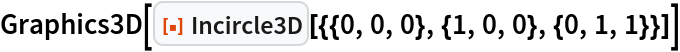Out=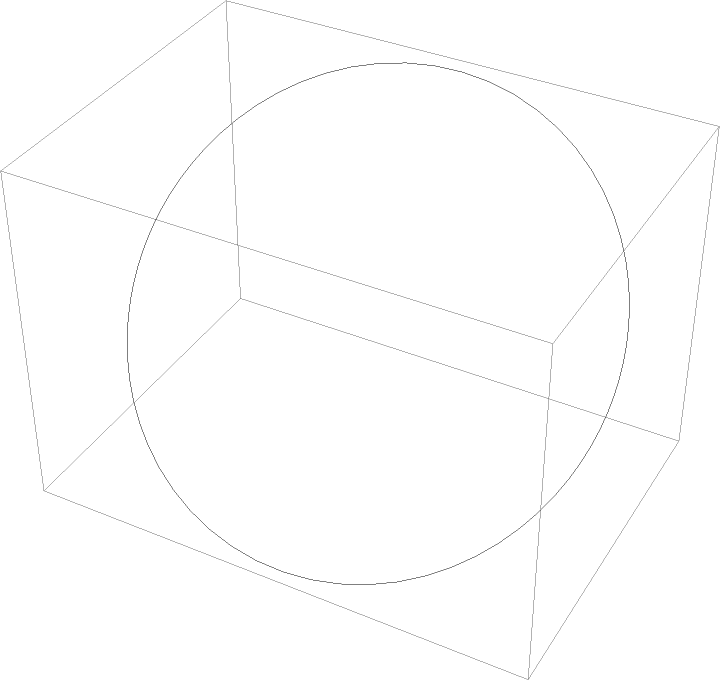### Scope (3)

Show a triangle and its incircle together:

 In:=Out=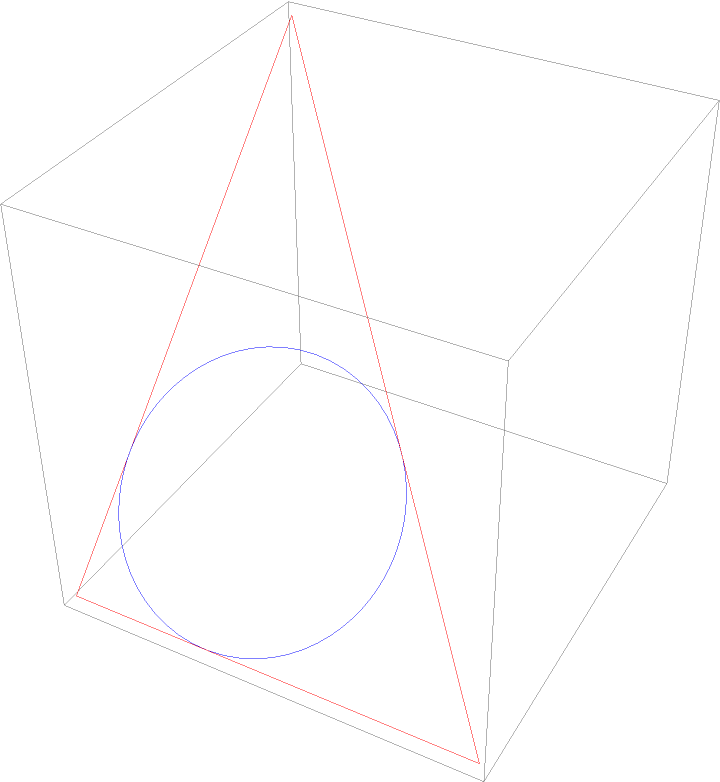Use Tube to render an incircle:

 In:=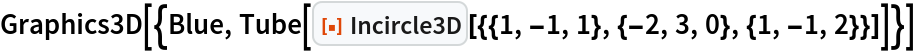Out=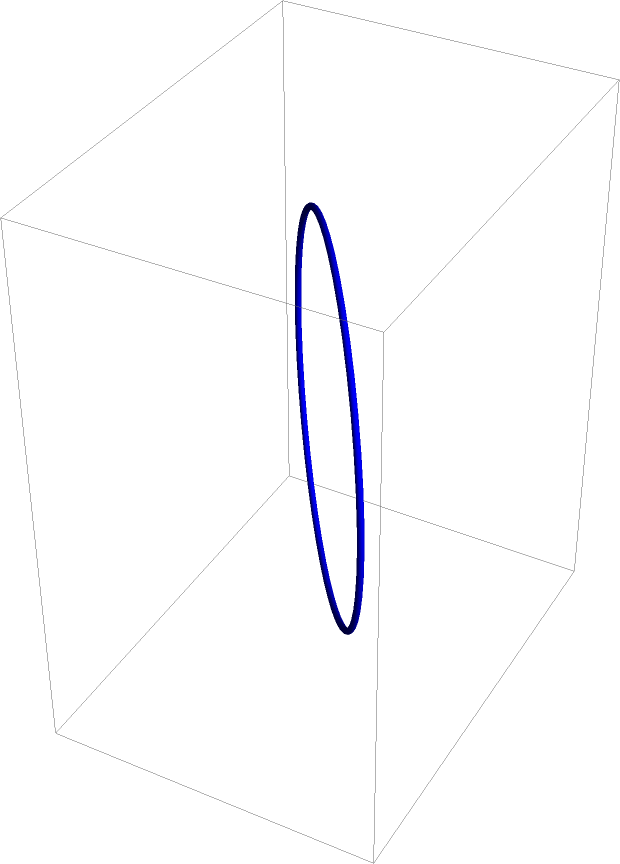In:=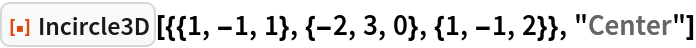Out=In:=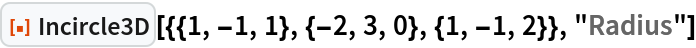Out=### Neat Examples (1)

Incircles of the faces of an icosahedron:

 In:=Out=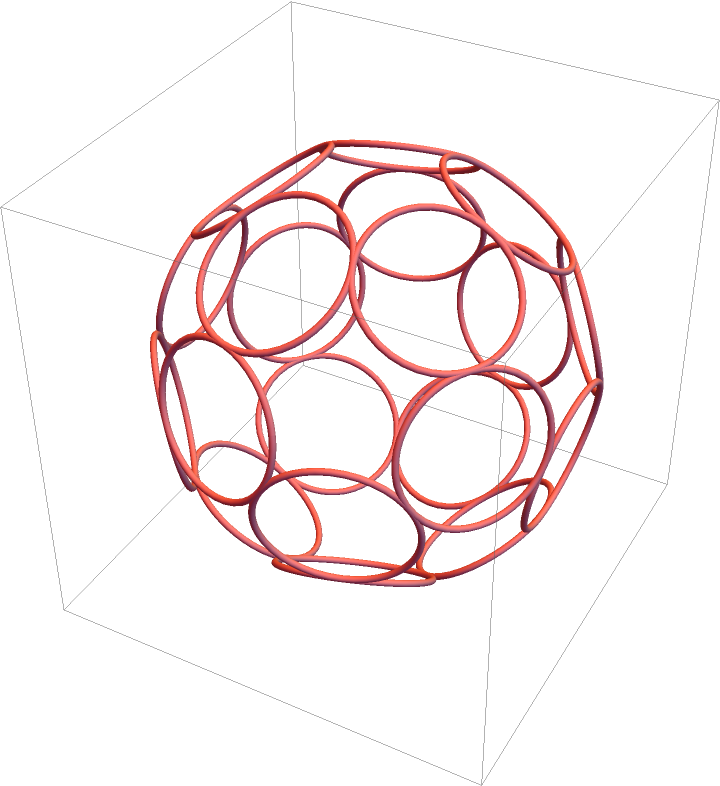## Version History

• 1.0.0 – 14 June 2021